# Effusion and Diffusion of Gases with Examples

Effusion and Diffusion of Gases with Examples

Diffusion:

Mixing molecules of one gas with molecules of another gas is called diffusion . Smell of a perfume or meal in a room are some common examples of diffusion of gases. Gases have different diffusion rates at different temperatures. Following formula shows ratio of diffusion rates of two gases at same temperature.

Diffusion rate (r) is directly proportional to average molecular velocity.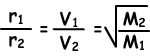Where; r1 and r2are diffusion rates of gas 1 and gas 2, V1 and V2 are average molecular velocities of gases and M1 and M2 are molecular masses of gases.

Equation given above is called also “Graham’s Diffusion Law”.

Now, we will give diffusion ratio of two different gases at different temperatures. Let T1 and T2 are absolute temperatures of gases.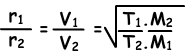To sum up;

Speed of gas diffusion;

• is inversely proportional to square root of molar mass
• is directly proportional to square root of absolute temperature

Thus;

If gases have same temperature, one of them having smaller molar mass has greater diffusion rate. If gases have equal molar mass, one of them having higher temperature has greater diffusion rate.

Effusion: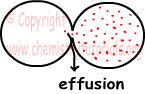As you can see from the picture given above, motion of the gases from one container to another by passing through small hole is called effusion (as given in the picture, in general second container is empty, or vacuum).Diffusion takes place under constant pressure on the contrary effusion takes place under pressure difference between containers. Effusion rates of gases changes according  to Graham’s diffusion law. Now we solve some examples related to diffusion and effusion of gases.

Example: If gases X and SO2 are send out at same time from points A and B, they meet at point 20 cm away from B. Which one of the following statements are true? (SO2=64)

I. Molar mass of X is 4

II. If we increase  absolute temperature of SO2 and keep temperature of X constant, meeting point of gases get closer to A.

III. If we decrease absolute temperatures of gases at same amount, meeting point does not change.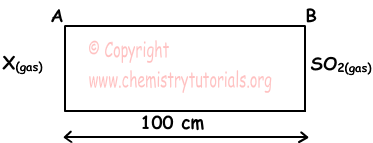Solution:

I. Since meeting point of gases is 20 cm away from B, molecules of X move 4 times faster than molecules of SO2.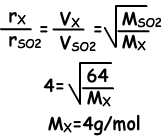I is true

II. SO2 moves slower than X, if we increase temperature of SO2, its speed increases and meeting point get closer point A. II is also true.

III. Decreasing temperatures of gases at same amount, does not affect meeting point. III is true

Example: Which one of the following statements are true for average molecular velocity of H2 and N2 molecules. (H=1, N=14)

I. N2 molecules at 40 0C are slower than H2 molecules at 40 0C.

II. H2 molecules at 80 0C are slower than N2 molecules at 40 0C.

III. N2 molecules at 80 0C are faster than H2 molecules at 40 0C.

Solution:

Molar mass of H2=2 g/mol, Molar mass of N2=28 g/mol.

I. Since molar mass of N2 is larger than H2, molecules of N2 move slower than H2. I is true

II. Average molecular speed is directly proportional to square root of absolute temperature. Thus, molecules of H2 are faster than molecules of N2. II is true

III. Molecules of N2 at 80 0C are faster than molecules of H2 at 40 0C. III is true.

Example: Under constant temperature, when we open the taps gases meet at point A. Find molecular mass of X(gas).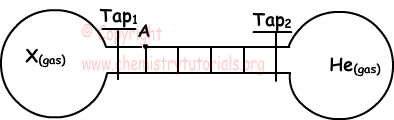Solution:

Since they meet at point A, molecules of He are faster than molecules of X.During same time He takes 4 unit distance, X takes 1 unit distance.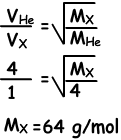Gases Exams and  Problem Solutions